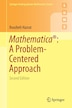Mathematica: A Problem-centered Approach

byRoozbeh Hazrat

Pricing and Purchase Info

\$53.66 online
\$62.50 list price save 14%
Earn 268 plum® points

Prices and offers may vary in store

Quantity:

In stock online

Ships free on orders over \$25

Not available in stores

Mathematica®: A Problem-Centered Approachintroduces the vast array of features and powerful mathematical functions of Mathematica using a multitude of clearly presented examples and worked- out problems. Each section starts with a description of a new topic and some basic examples. The author then demonstrates the use of new commands through three categories of problems

- the first category highlights those essential parts of the text that demonstrate the use of new commands in Mathematica whilst solving each problem presented;

- the second comprises problems that further demonstrate the use of commands previously introduced to tackle different situations; and

- the third presents more challenging problems for further study.

The intention is to enable the reader to learn from the codes, thus avoiding long and exhausting explanations.

While based on a computer algebra course taught to undergraduate students of mathematics, science, engineering and finance, the book also includes chapters on calculus and solving equations, and graphics, thus covering all the basic topics in Mathematica. With its strong focus upon programming and problem solving, and an emphasis on using numerical problems that do not need any particular background in mathematics, this book is also ideal for self-study and as an introduction to researchers who wish to use Mathematica as a computational tool.

Mathematica®: A Problem-Centered Approachcomes with a free 30 day trial of the Wolfram Mathematica(R) software'

Roozbeh Hazrat obtained his PhD in Pure Mathematics from the University of Bielefeld, Germany in 2002. He did research at the Australian National University, the Max Planck Institute in Bonn and the City University of New York. He was a Reader (Associate Professor) in Pure Mathematics at Queen's University Belfast, United Kingdom, wher...
Title:Mathematica: A Problem-centered ApproachFormat:PaperbackDimensions:318 pages, 23.5 × 15.5 × 0.02 inPublished:January 12, 2016Publisher:Springer-Verlag/Sci-Tech/TradeLanguage:English

The following ISBNs are associated with this title:

ISBN - 10:3319275844

ISBN - 13:9783319275840

Reviews

From the Author

Mathematica®: A Problem-Centered Approachintroduces the vast array of features and powerful mathematical functions of Mathematica using a multitude of clearly presented examples and worked- out problems. Each section starts with a description of a new topic and some basic examples. The author then demonstrates the use of new commands through three categories of problems- the first category highlights those essential parts of the text that demonstrate the use of new commands in Mathematica whilst solving each problem presented;- the second comprises problems that further demonstrate the use of commands previously introduced to tackle different situations; and- the third presents more challenging problems for further study.The intention is to enable the reader to learn from the codes, thus avoiding long and exhausting explanations.While based on a computer algebra course taught to undergraduate students of mathematics, science, engineering and finance, the book also includes chapters on calculus and solving equations, and graphics, thus covering all the basic topics in Mathematica. With its strong focus upon programming and problem solving, and an emphasis on using numerical problems that do not need any particular background in mathematics, this book is also ideal for self-study and as an introduction to researchers who wish to use Mathematica as a computational tool.Mathematica®: A Problem-Centered Approachcomes with a free 30 day trial of the Wolfram Mathematica(R) software'